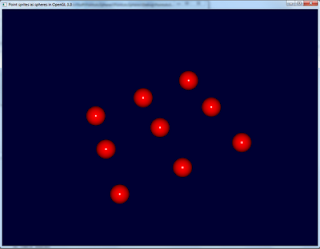## Tuesday, January 25, 2011

### Point sprites as spheres in OpenGL3.3

Someone asked on opengl.org forums to generate sphere without any loop. It is possible to do so using particle sprites. The bulk of the stuff goes in the fragment shader which is as follows,

` #version 330out vec4 vFragColor;uniform vec3 Color;uniform vec3 lightDir;void main(void){    // calculate normal from texture coordinates    vec3 N;    N.xy = gl_PointCoord* 2.0 - vec2(1.0);        float mag = dot(N.xy, N.xy);    if (mag > 1.0) discard;   // kill pixels outside circle    N.z = sqrt(1.0-mag);    // calculate lighting    float diffuse = max(0.0, dot(lightDir, N));    vFragColor = vec4(Color,1) * diffuse;}`

which first gets the normal from the texture coordinates. Then, it does a dot product of the normal with the light vector. Adding specular component should be trivial. This gives the following output. You may download the source code from hereAdding in the specular term gives even better result. Have a look at this image.This image was generated using the following shader.
`#version 330out vec4 vFragColor;uniform vec3 Color;uniform vec3 lightDir;float Ns = 250;vec4 mat_specular=vec4(1); vec4 light_specular=vec4(1); void main(void){    // calculate normal from texture coordinates    vec3 N;    N.xy = gl_PointCoord* 2.0 - vec2(1.0);        float mag = dot(N.xy, N.xy);    if (mag > 1.0) discard;   // kill pixels outside circle    N.z = sqrt(1.0-mag);    // calculate lighting    float diffuse = max(0.0, dot(lightDir, N));     vec3 eye = vec3 (0.0, 0.0, 1.0);    vec3 halfVector = normalize( eye + lightDir);    float spec = max( pow(dot(N,halfVector), Ns), 0.);     vec4 S = light_specular*mat_specular* spec;    vFragColor = vec4(Color,1) * diffuse + S;}`

The spheres rendered this way will not change their size when we zoom in and out. I will show in a later blog entry how to do that by setting the point size in the vertex shader.